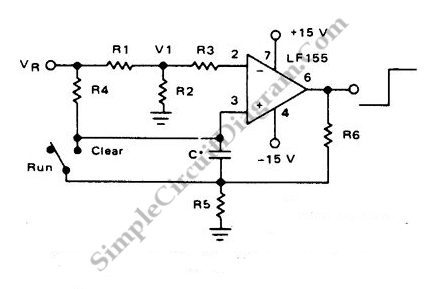# Long Interval RC Timer Using Op-Amp

With the help of high gain high impedance operational amplifier, we can build a long time delay with resistor-capacitor (RC) circuit since it allow high resistance resistors to be used. We can calculate the time (t) using the following formula; Time (t) = R4.C.ln(Vr/(Vr-Vi)). For design example is a 100 second timer, where this circuit uses this following components: Vr=10V, C=1uF, R3=R4=144M, R6=20K, R5=2k, R1=R2=1k.